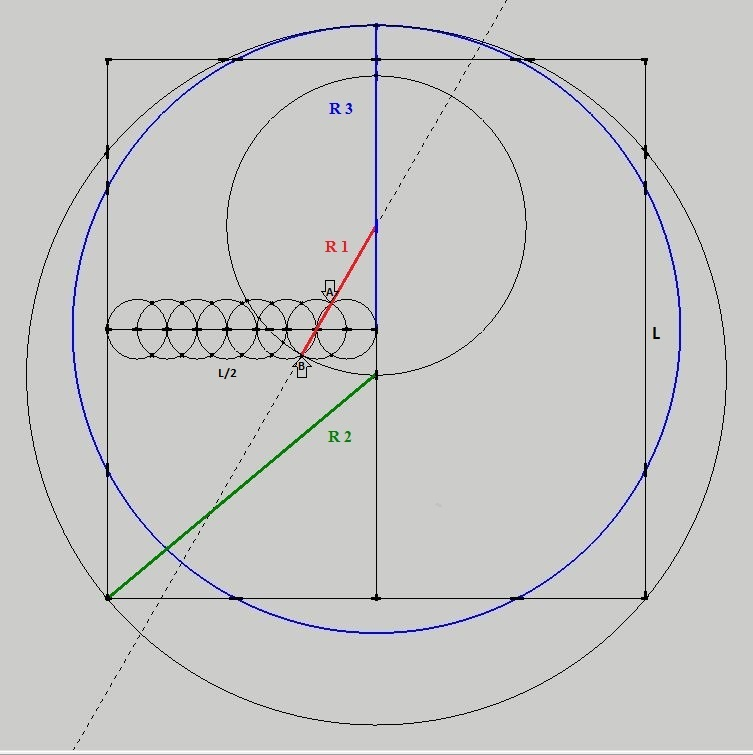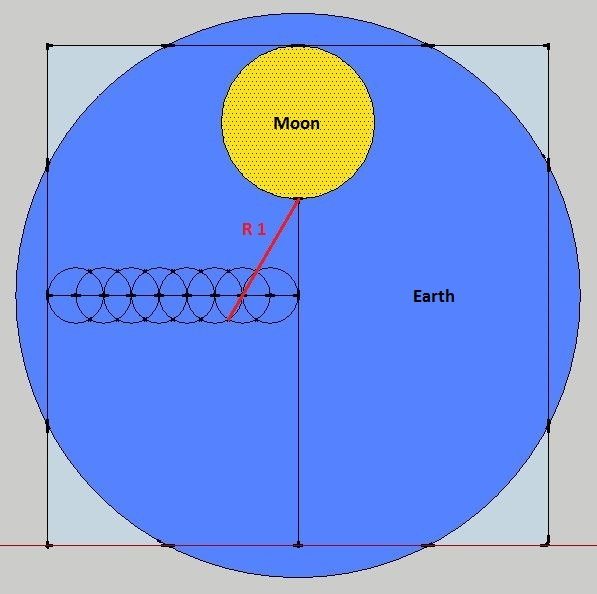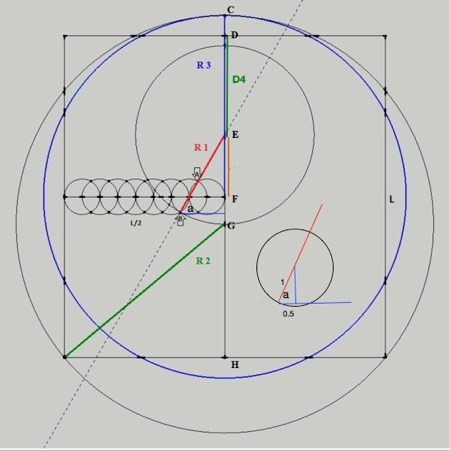Home | Study Links | Rhymes |

## Squaring the Circle method shows a close Earth Moon Ratio

This simple method of squaring the circle (an attempt to make a circle equal in area to that of a
given square or visa versa) using a straightedge and compass, has an accuracy that exceeds 99.9%.1. Place eight interlocking circles along a straight line (L/2)

2. Create the square (L2)3. Draw a line through points A and B and circle R1 as shown

4. Circle R2

5. Circle R3 which is the radius

In this square the circle method the diameter ratio Earth / Moon is 3.66... It matches the real thing!Thank you Michael Joyce for putting this into proper mathematical format for me.

"Here follows a mathematical proof of Gertjan’s discovery.

If the radius of each of the small 8 circles is 1, then the square will have sides of length 18.
D4 represents the diameter of a circle, R1, R2, R3 the radii of other circles.1- To calculate angle “a”
The diagram in the lower right quadrant shows an enlargement of the second small circle
cosine a           = 0.5 divided by 1
= 1/2
Therefore a     = 60 degrees.

2- To calculate the length R1
cosine a           = 2 divided by (R1 - 1)
therefore R1    = 5

3- To calculate the length R2 using Pythagoras’ theorem
R2²        = GH² + 92
GH         = 18 – DG
GH         = 9 - FG

sin 60°  = EF divided by 4
EF         = 4 x sin 60°           = 3.4641016151
FG         = EG(R1) - EF          = 1.5358983848
GH        = 9 - 1.535898384    = 7.4641016151

R22        =  GH² + 92
= (7.4641016151)2 + 81
= 136.7128129211
R2         = square root (136.7128129211)
= 11.6924254507

4- To calculate the length R3
R3         = R2 - FG
= 11.6924254507 - 1.5358983848
= 10.1565270658

Squaring the Circle:
Area of square = 18 x 18                                                       = 324
Area of circle   = pi x R32 = 3.141… x 10.1565270658 2           = 324.071122253... (Q.E.D)

5- To calculate the length D4
D4         = DF - 4 x sin 60°
= 9 - 3.4641016151
= 5.5358983848

Earth : Moon diameters
R3 (10.1565270658 x 2) : D4 (5.5358983848) or (20.3130541317 : 5.5358983848)
Or 3.66933 : 1

The ratio of diameters Earth : Moon as 7920 : 2160 (11:3)
Or 3.66666 : 1

Answers are the same with negligible error ‼"

Since the Earth and Moon are only approximately spherical; no single value can serve as a
definitive radius. However, the Earth and Moon will be exact spheres in an absolute reality.
Perhaps with ratio of diameters= 3.66943... : 1